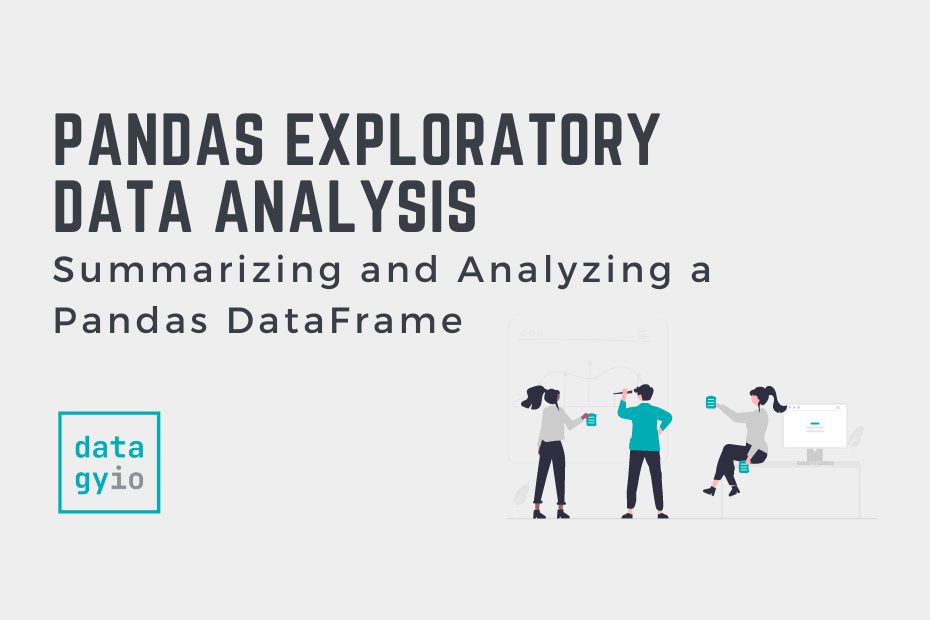# Pandas## Summarizing and Analyzing a Pandas DataFrame

In this tutorial, you’ll learn how to quickly summarize and analyze a Pandas DataFrame. By the end of this tutorial, you’ll have learned to take on some exploratory analysis of your dataset using pandas. You’ll learn how to calculate general… Read More »Summarizing and Analyzing a Pandas DataFrame## Transforming Pandas Columns with map and apply

In this tutorial, you’ll learn how to transform your Pandas DataFrame columns using vectorized functions and custom functions using the map and apply methods. By the end of this tutorial, you’ll have a strong understanding of how Pandas applies vectorized… Read More »Transforming Pandas Columns with map and apply## Working with External Libraries in Python

In this tutorial, you’ll learn how to work with external libraries in Python. By the end of this tutorial, you’ll have learned why external libraries are essential to your Python programming journey, and how to install and import external libraries.… Read More »Working with External Libraries in Python## Counting Values in Pandas with value_counts

In this tutorial, you’ll learn how to use the Pandas value_counts method to count values in your DataFrame and to create frequency tables. Being able to count values in your dataset is an important step in better understanding the distribution… Read More »Counting Values in Pandas with value_counts## Binning Data in Pandas with cut and qcut

In this tutorial, you’ll learn how to bin data in Python with the Pandas cut and qcut functions. You’ll learn why binning is a useful skill in Pandas and how you can use it to better group and distill information.… Read More »Binning Data in Pandas with cut and qcut## DateTime in Pandas and Python

In this tutorial, you’ll learn how to work with dates, times, and DateTime in Pandas and Python. Working with DateTime in Python and Pandas can be a complicated thing. This guide aims to make the complicated, simple, by focusing on… Read More »DateTime in Pandas and Python## Plotting in Python with Matplotlib

In this tutorial, you’ll learn how to get started with plotting in Python with the matplotlib library. You’ll learn how the matplotlib library works and gain an understanding of its “anatomy”. You’ll learn how to plot and customize some simple… Read More »Plotting in Python with Matplotlib## Data Cleaning and Preparation in Pandas and Python

In this tutorial, you’ll learn how to clean and prepare data in a Pandas DataFrame. You’ll learn how to work with missing data, how to work with duplicate data, and dealing with messy string data. Being able to effectively clean… Read More »Data Cleaning and Preparation in Pandas and Python## Pandas GroupBy: Group, Summarize, and Aggregate Data in Python

The Pandas groupby method is an incredibly powerful tool to help you gain effective and impactful insight into your dataset. In just a few, easy to understand lines of code, you can aggregate your data in incredibly straightforward and powerful… Read More »Pandas GroupBy: Group, Summarize, and Aggregate Data in Python## Pandas Datetime to Date Parts (Month, Year, etc.)

In this tutorial, you’ll learn how to use Pandas to extract date parts from a datetime column, such as to date, year, and month. Pandas provides a number of easy ways to extract parts from a datetime object, including using… Read More »Pandas Datetime to Date Parts (Month, Year, etc.)## Calculate the Pearson Correlation Coefficient in Python

In this tutorial, you’ll learn how to calculate the Pearson Correlation Coefficient in Python. The tutorial will cover a brief recap of what the Pearson correlation coefficient is, how to calculate it with SciPy and how to calculate it for… Read More »Calculate the Pearson Correlation Coefficient in Python## Python Dictionaries: A Complete Overview

Python dictionaries are an incredibly useful data type, which allow you to store data in key:value pairs. In this tutorial, you’ll learn all you need to know to get up and running with Python dictionaries, including: The basics of creating… Read More »Python Dictionaries: A Complete Overview